geom_rect() and geom_tile() do the same thing, but are parameterised differently: geom_rect() uses the locations of the four corners (xmin, xmax, ymin and ymax), while geom_tile() uses the center of the tile and its size (x, y, width, height). geom_raster() is a high performance special case for when all the tiles are the same size.

## Usage

geom_raster(
mapping = NULL,
data = NULL,
stat = "identity",
position = "identity",
...,
hjust = 0.5,
vjust = 0.5,
interpolate = FALSE,
na.rm = FALSE,
show.legend = NA,
inherit.aes = TRUE
)

geom_rect(
mapping = NULL,
data = NULL,
stat = "identity",
position = "identity",
...,
linejoin = "mitre",
na.rm = FALSE,
show.legend = NA,
inherit.aes = TRUE
)

geom_tile(
mapping = NULL,
data = NULL,
stat = "identity",
position = "identity",
...,
linejoin = "mitre",
na.rm = FALSE,
show.legend = NA,
inherit.aes = TRUE
)

## Arguments

mapping

Set of aesthetic mappings created by aes(). If specified and inherit.aes = TRUE (the default), it is combined with the default mapping at the top level of the plot. You must supply mapping if there is no plot mapping.

data

The data to be displayed in this layer. There are three options:

If NULL, the default, the data is inherited from the plot data as specified in the call to ggplot().

A data.frame, or other object, will override the plot data. All objects will be fortified to produce a data frame. See fortify() for which variables will be created.

A function will be called with a single argument, the plot data. The return value must be a data.frame, and will be used as the layer data. A function can be created from a formula (e.g. ~ head(.x, 10)).

stat

The statistical transformation to use on the data for this layer, either as a ggproto Geom subclass or as a string naming the stat stripped of the stat_ prefix (e.g. "count" rather than "stat_count")

position

Position adjustment, either as a string naming the adjustment (e.g. "jitter" to use position_jitter), or the result of a call to a position adjustment function. Use the latter if you need to change the settings of the adjustment.

...

Other arguments passed on to layer(). These are often aesthetics, used to set an aesthetic to a fixed value, like colour = "red" or size = 3. They may also be parameters to the paired geom/stat.

hjust, vjust

horizontal and vertical justification of the grob. Each justification value should be a number between 0 and 1. Defaults to 0.5 for both, centering each pixel over its data location.

interpolate

If TRUE interpolate linearly, if FALSE (the default) don't interpolate.

na.rm

If FALSE, the default, missing values are removed with a warning. If TRUE, missing values are silently removed.

show.legend

logical. Should this layer be included in the legends? NA, the default, includes if any aesthetics are mapped. FALSE never includes, and TRUE always includes. It can also be a named logical vector to finely select the aesthetics to display.

inherit.aes

If FALSE, overrides the default aesthetics, rather than combining with them. This is most useful for helper functions that define both data and aesthetics and shouldn't inherit behaviour from the default plot specification, e.g. borders().

linejoin

Line join style (round, mitre, bevel).

## Details

geom_rect() and geom_tile()'s respond differently to scale transformations due to their parameterisation. In geom_rect(), the scale transformation is applied to the corners of the rectangles. In geom_tile(), the transformation is applied only to the centres and its size is determined after transformation.

## Aesthetics

geom_tile() understands the following aesthetics (required aesthetics are in bold):

• x

• y

• alpha

• colour

• fill

• group

• height

• linetype

• linewidth

• width

Note that geom_raster() ignores colour.

Learn more about setting these aesthetics in vignette("ggplot2-specs").

## Examples

# The most common use for rectangles is to draw a surface. You always want
# to use geom_raster here because it's so much faster, and produces
# smaller output when saving to PDF
ggplot(faithfuld, aes(waiting, eruptions)) +
geom_raster(aes(fill = density))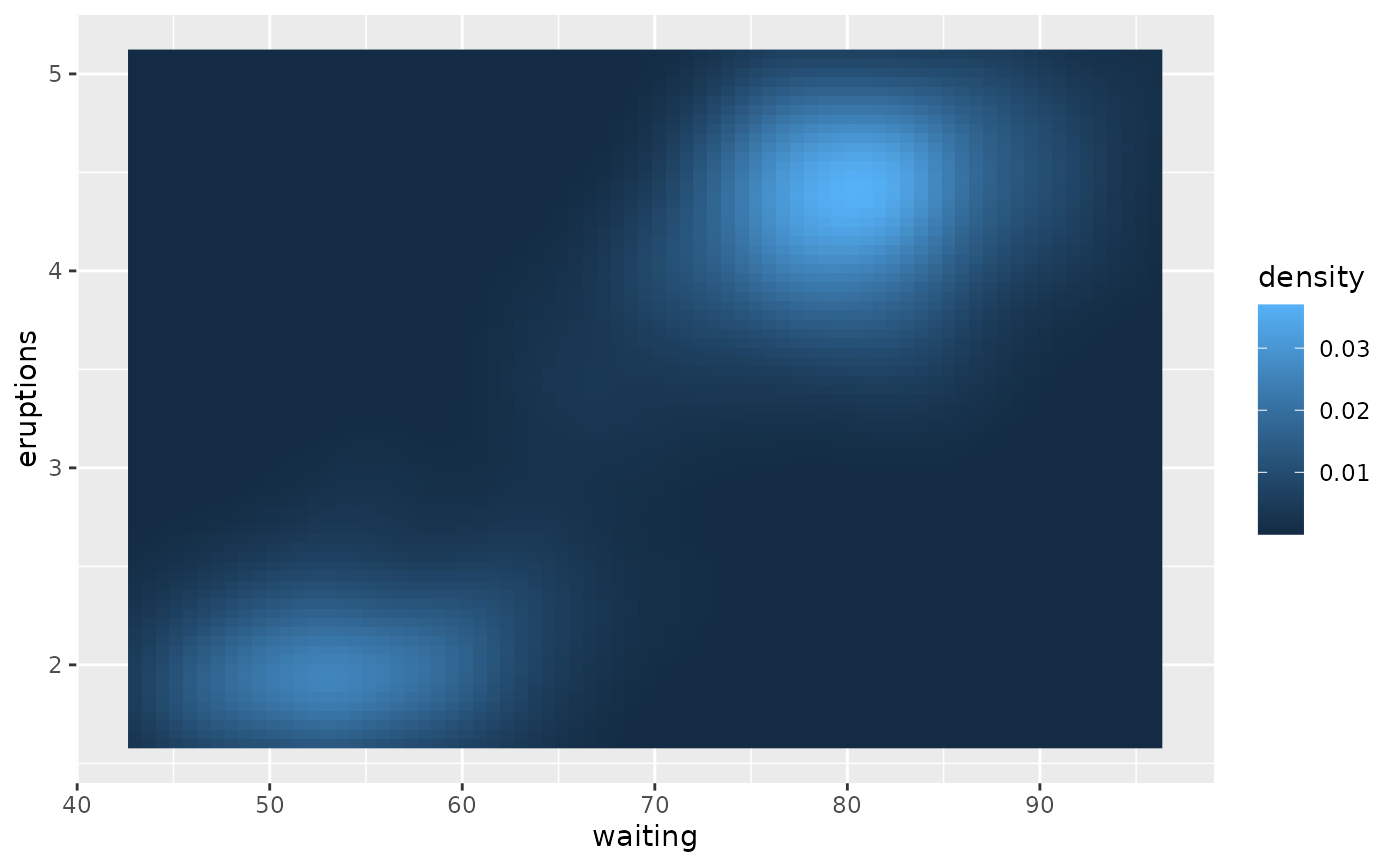# Interpolation smooths the surface & is most helpful when rendering images.
ggplot(faithfuld, aes(waiting, eruptions)) +
geom_raster(aes(fill = density), interpolate = TRUE)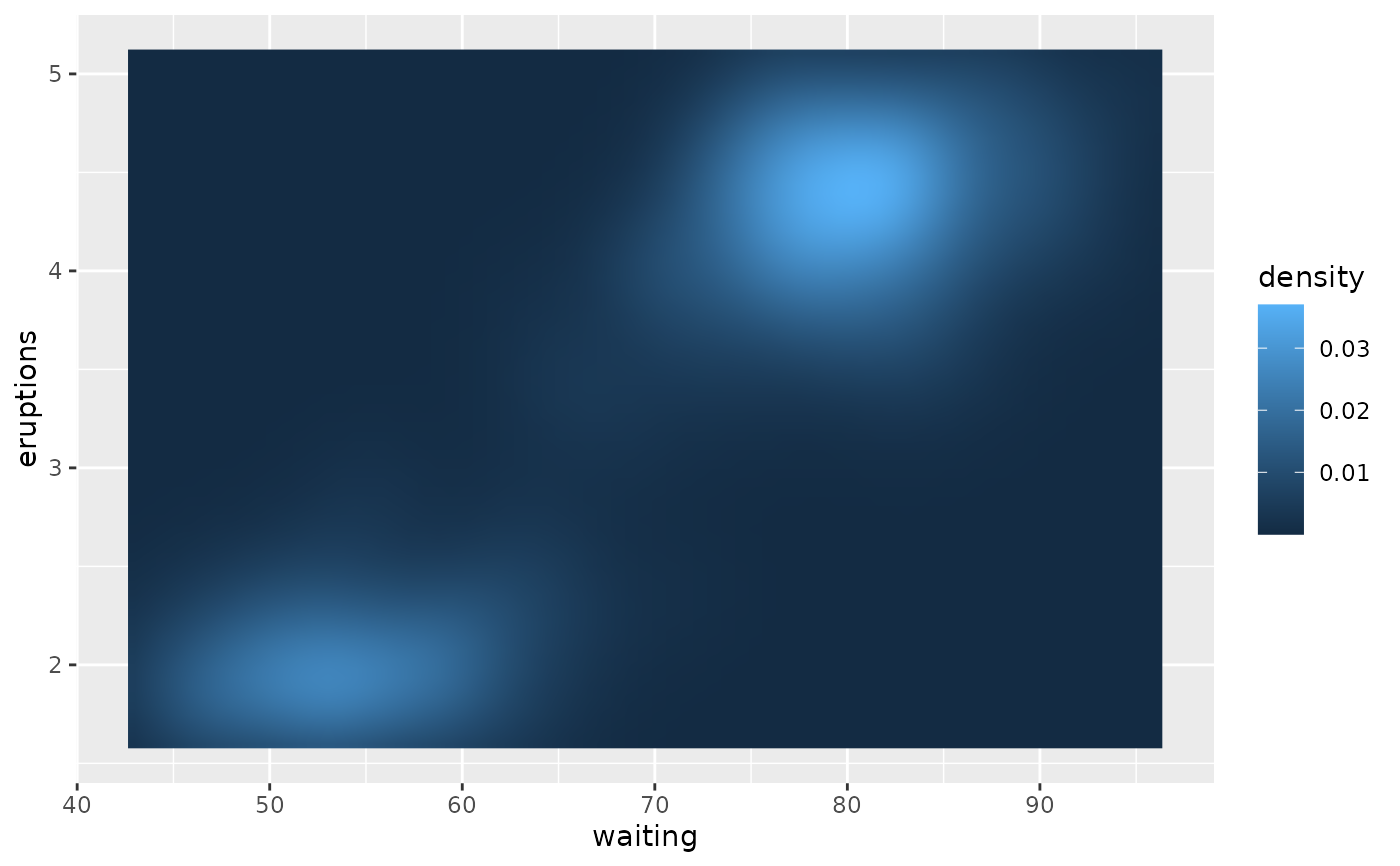# If you want to draw arbitrary rectangles, use geom_tile() or geom_rect()
df <- data.frame(
x = rep(c(2, 5, 7, 9, 12), 2),
y = rep(c(1, 2), each = 5),
z = factor(rep(1:5, each = 2)),
w = rep(diff(c(0, 4, 6, 8, 10, 14)), 2)
)
ggplot(df, aes(x, y)) +
geom_tile(aes(fill = z), colour = "grey50")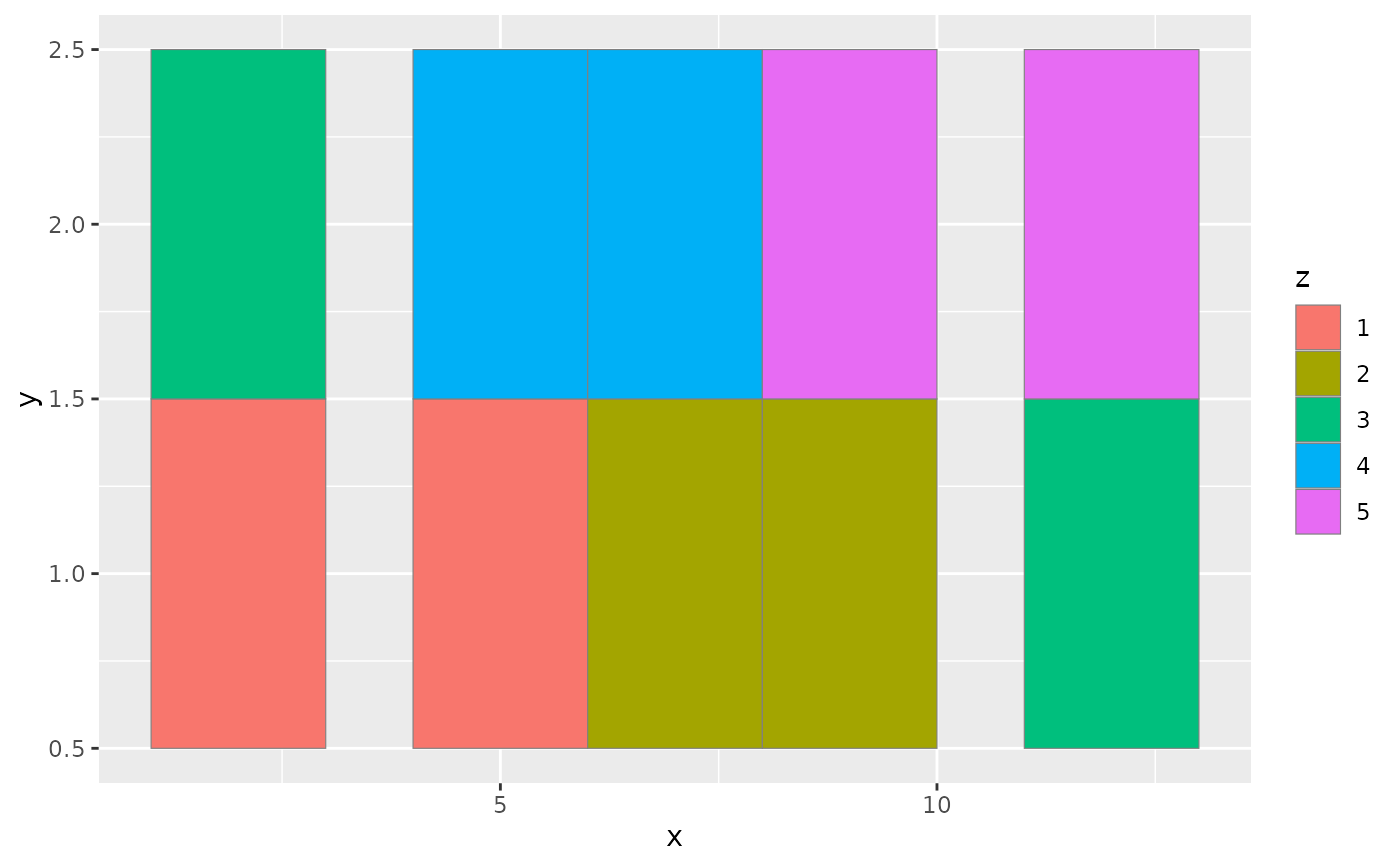ggplot(df, aes(x, y, width = w)) +
geom_tile(aes(fill = z), colour = "grey50")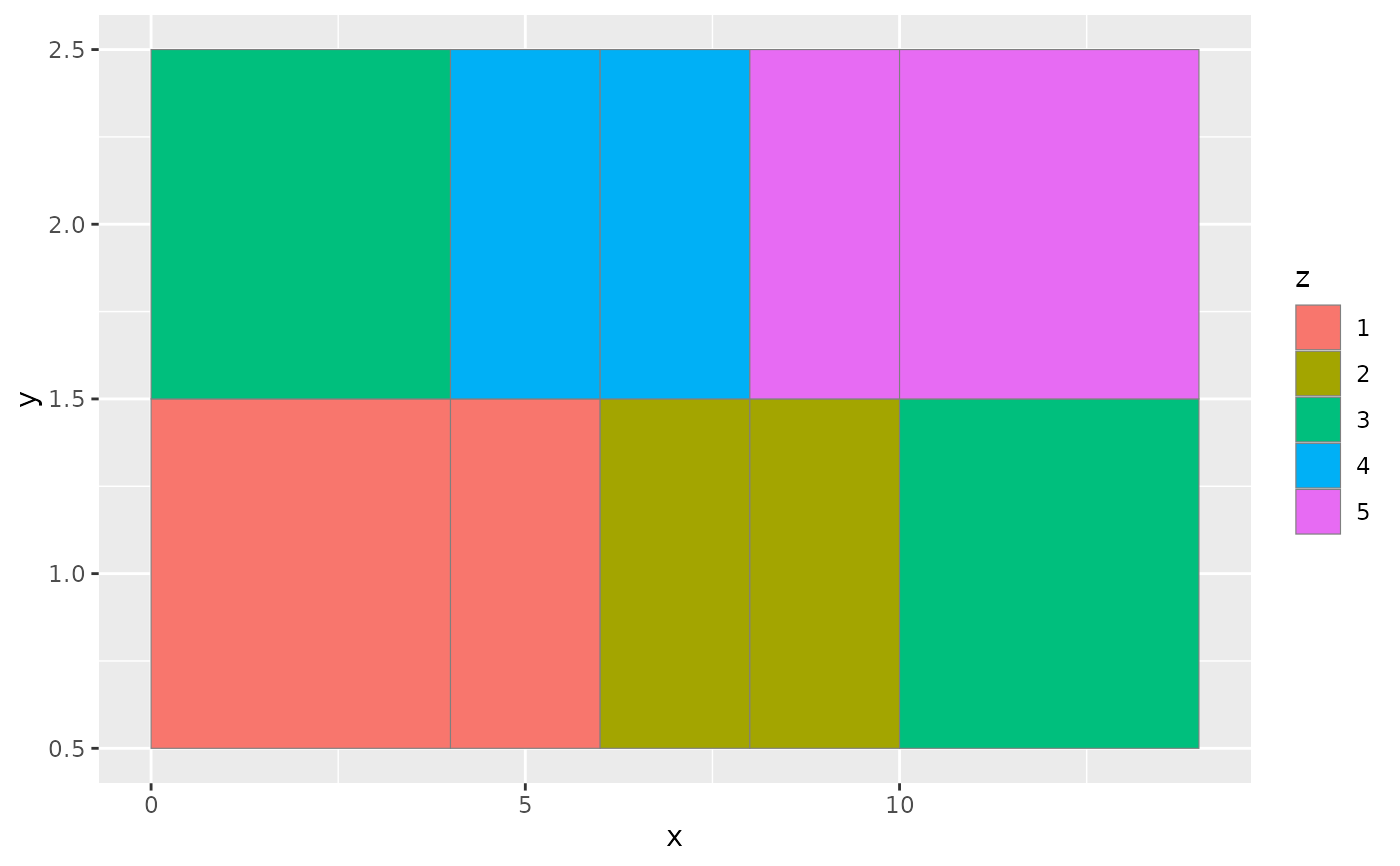ggplot(df, aes(xmin = x - w / 2, xmax = x + w / 2, ymin = y, ymax = y + 1)) +
geom_rect(aes(fill = z), colour = "grey50")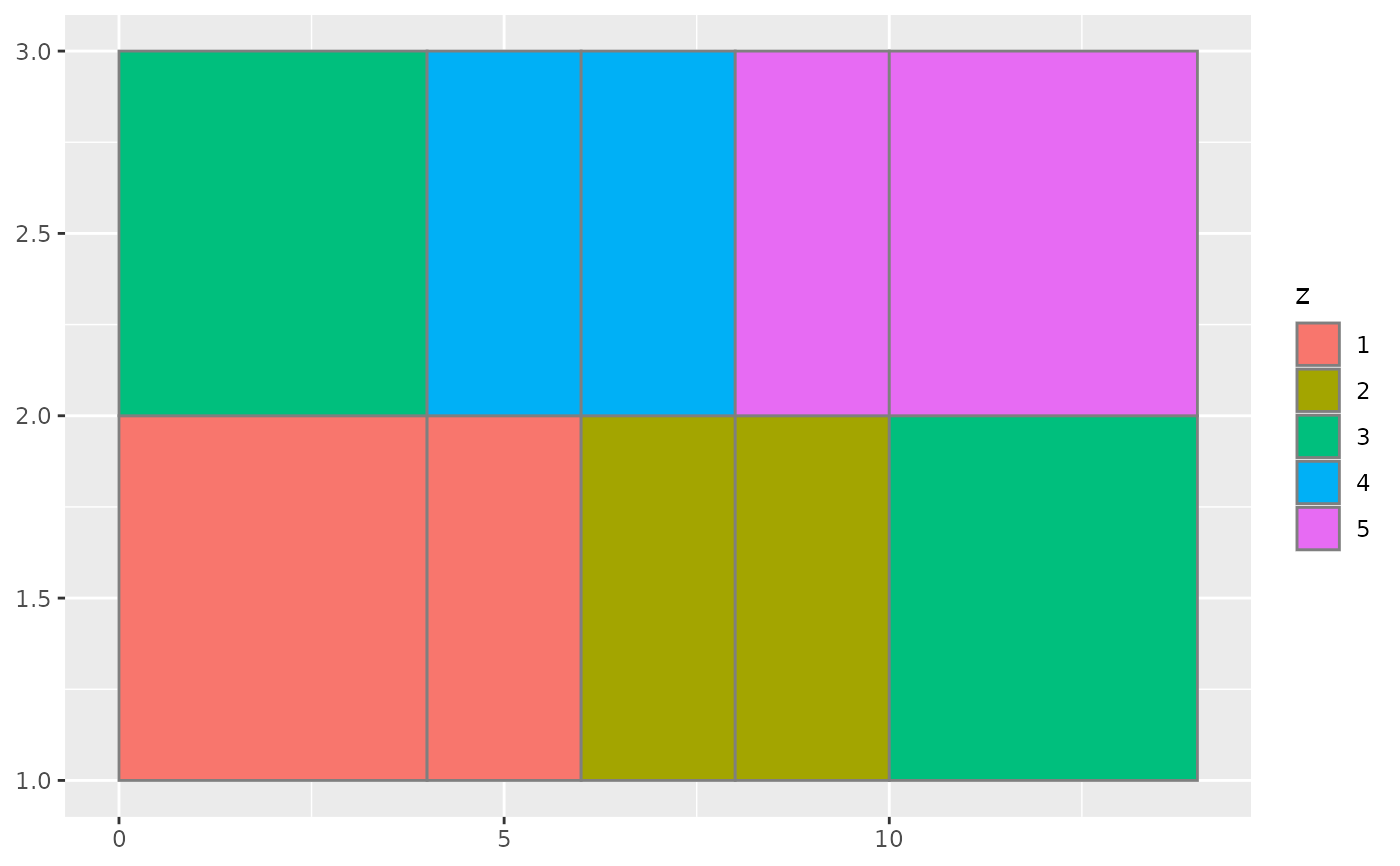# \donttest{
# Justification controls where the cells are anchored
df <- expand.grid(x = 0:5, y = 0:5)
set.seed(1)
df\$z <- runif(nrow(df))
# default is compatible with geom_tile()
ggplot(df, aes(x, y, fill = z)) +
geom_raster()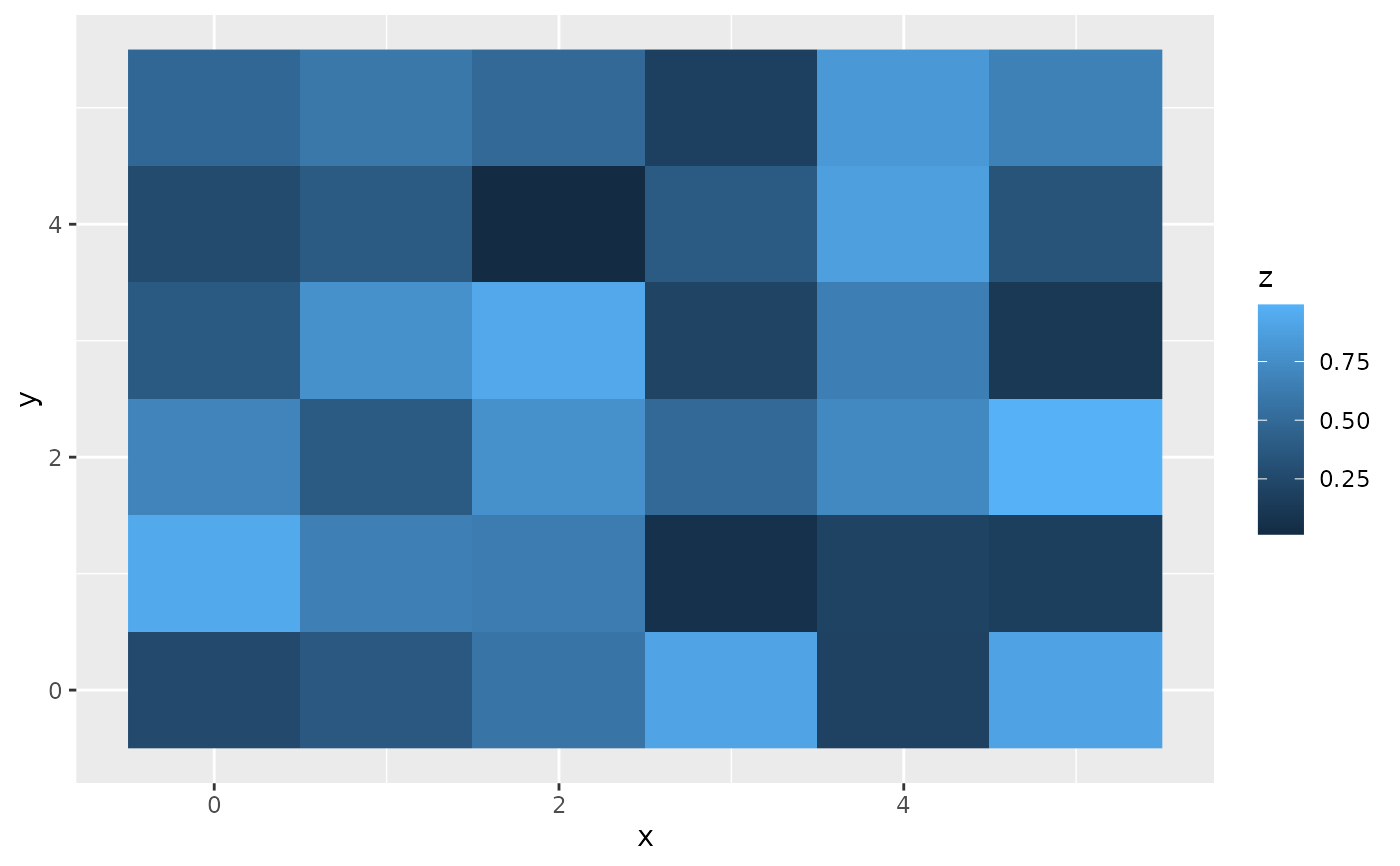ggplot(df, aes(x, y, fill = z)) +
geom_raster(hjust = 0, vjust = 0)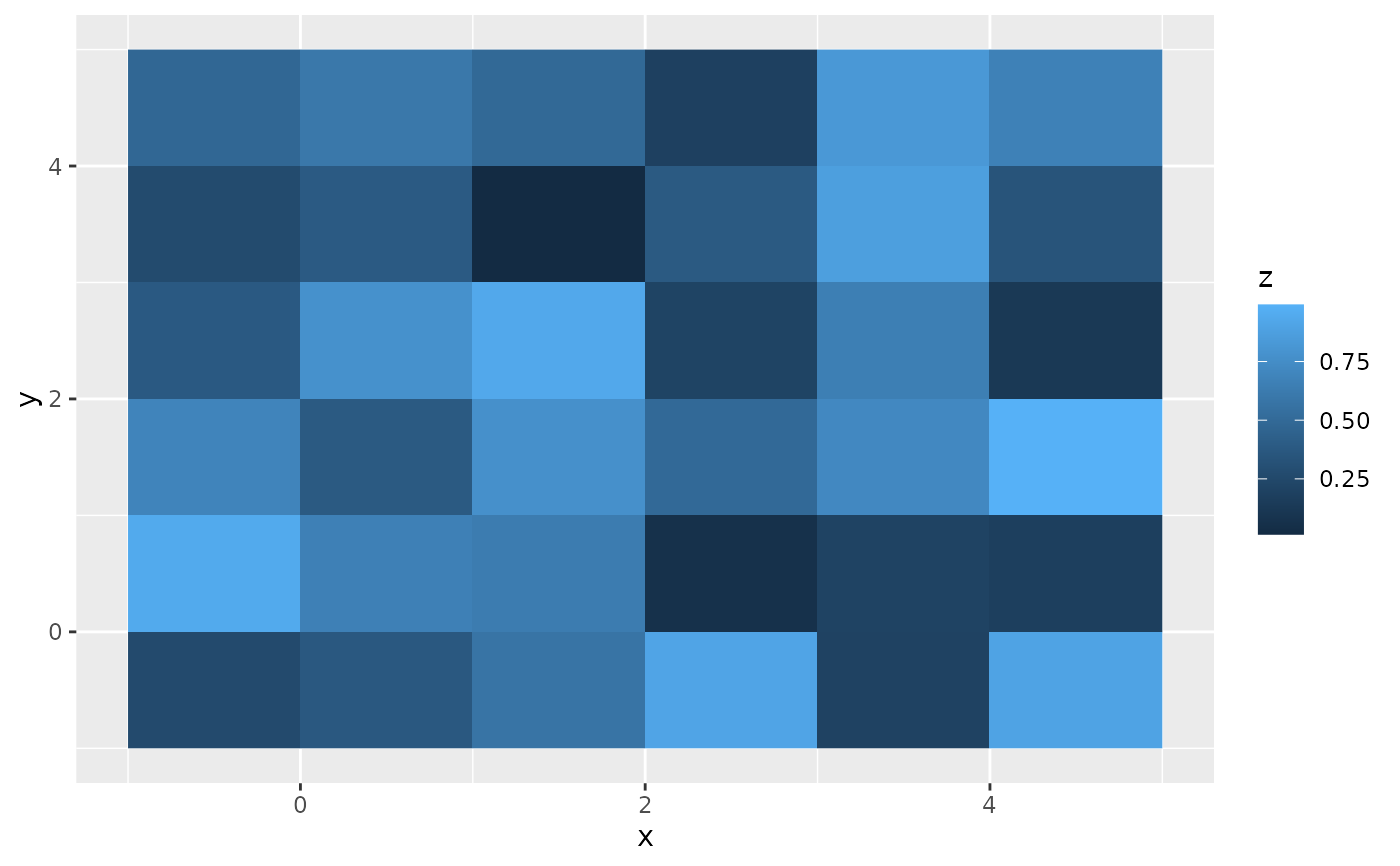# Inspired by the image-density plots of Ken Knoblauch
cars <- ggplot(mtcars, aes(mpg, factor(cyl)))
cars + geom_point()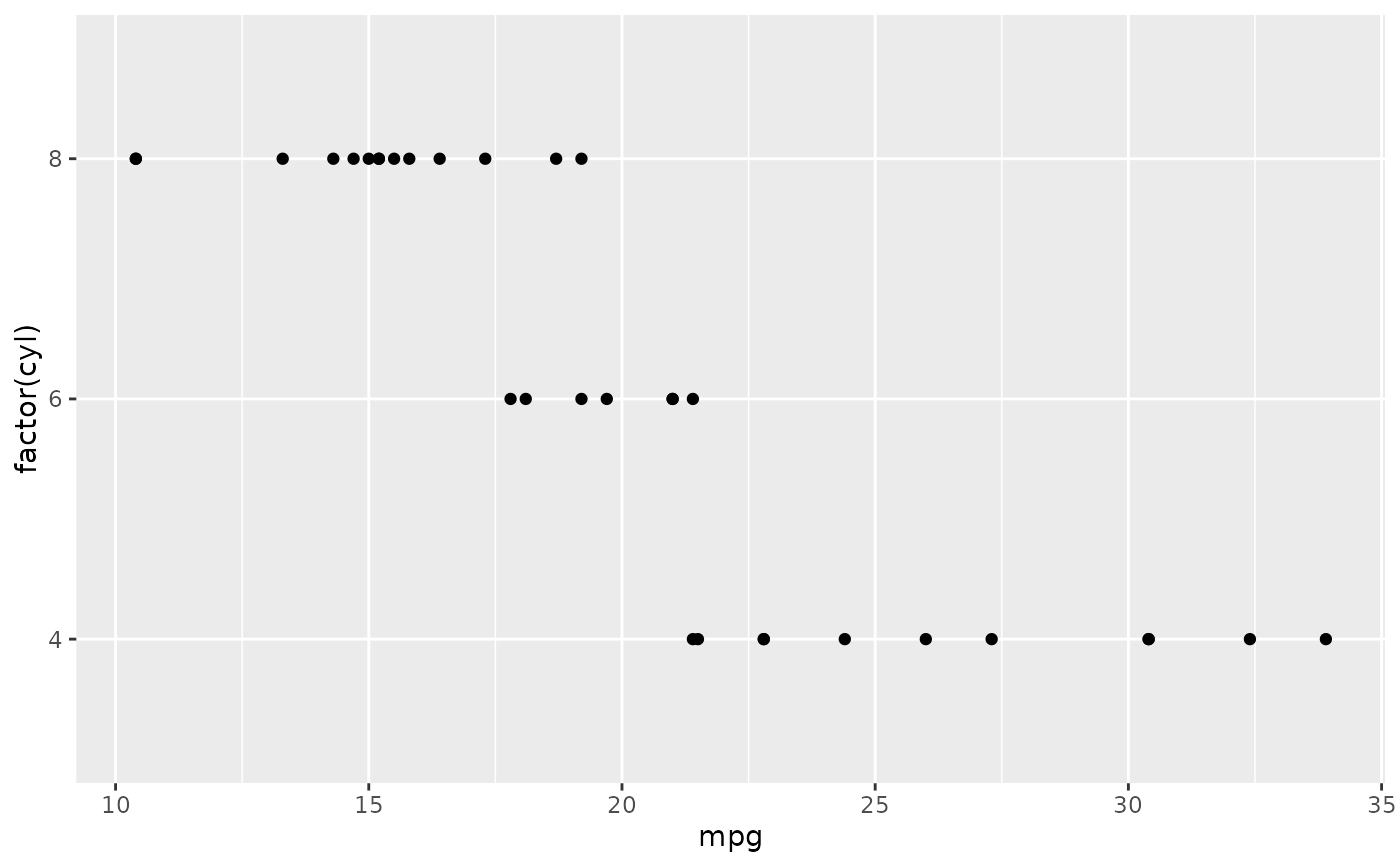cars + stat_bin2d(aes(fill = after_stat(count)), binwidth = c(3,1))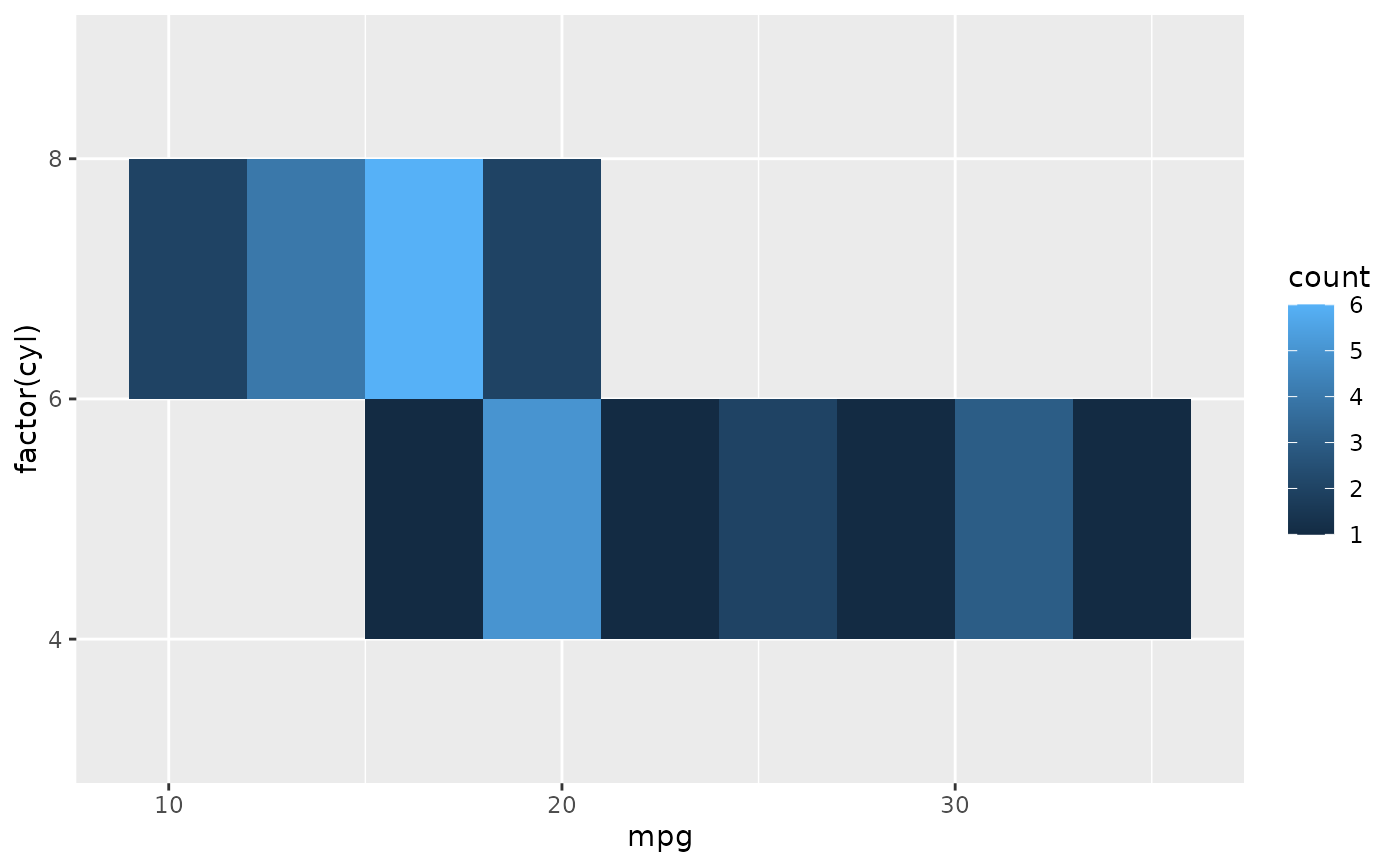cars + stat_bin2d(aes(fill = after_stat(density)), binwidth = c(3,1))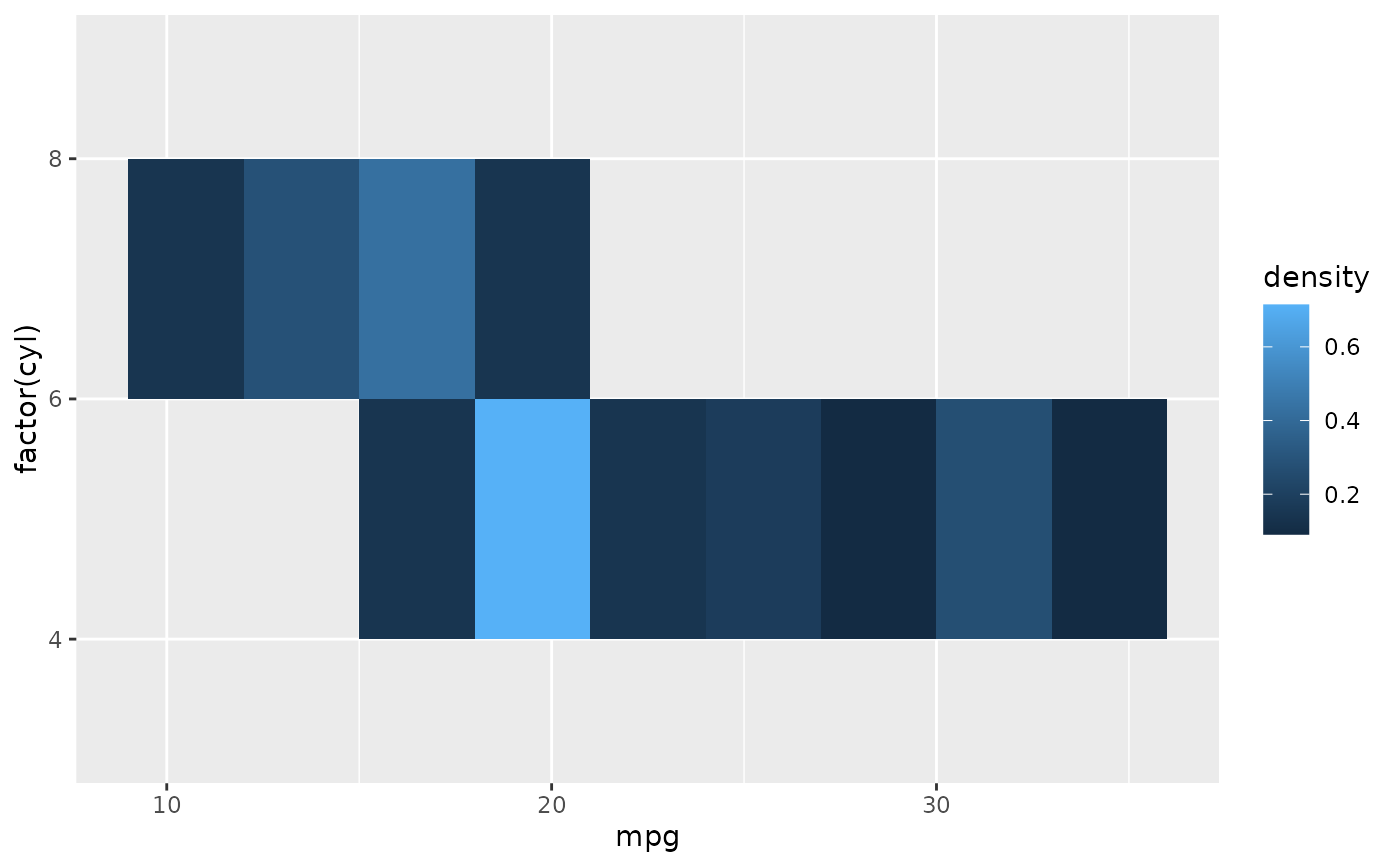cars +
stat_density(
aes(fill = after_stat(density)),
geom = "raster",
position = "identity"
)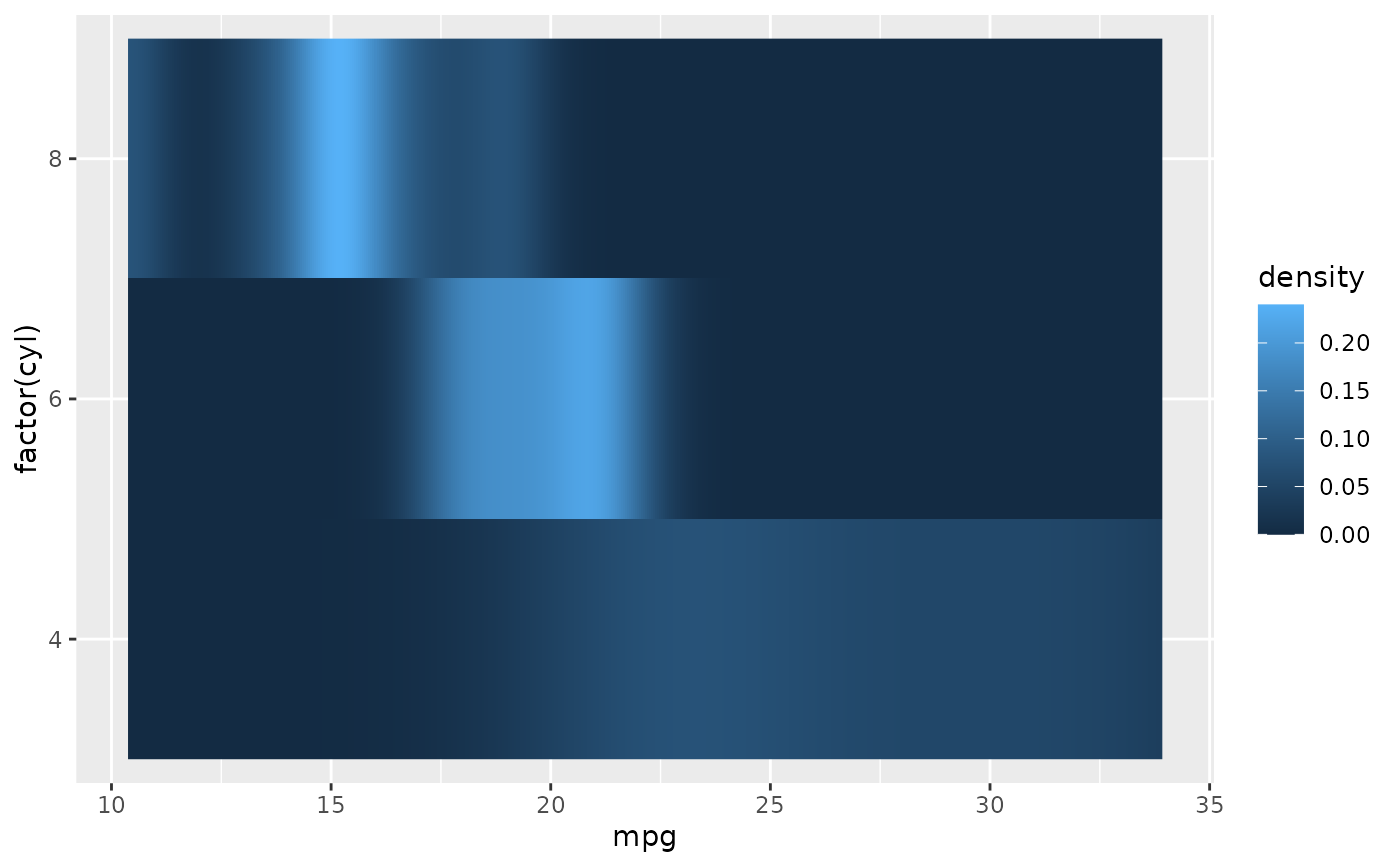cars +
stat_density(
aes(fill = after_stat(count)),
geom = "raster",
position = "identity"
)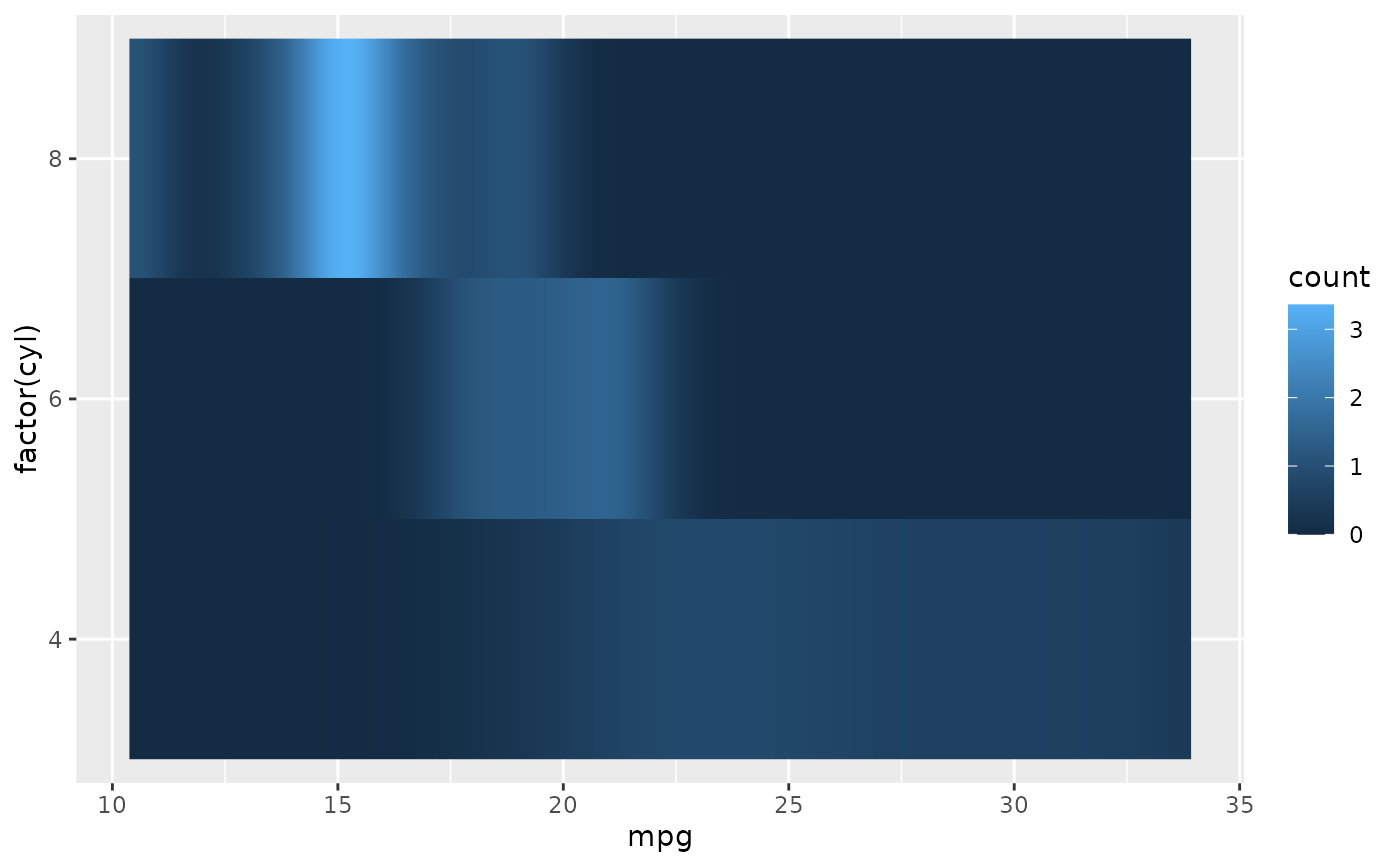# }